General Article

International Journal of Sustainable Building Technology and Urban Development. 30 September 2023. 389-404
https://doi.org/10.22712/susb.20230029

# MAIN

• Introduction

•   IS 800-2007 Code provision for calculation of strength in block shear mode of failure

•   Eurocode 3 Specification for Block Tearing

•   AISC 2005 Specification for Block Shear:

•   CSA-S16-09 – Design of Steel Structures

• Research Objectives and Motivation

• Previous Studies

• Current Study : State-of-the-art FEA models for tension members with bolted connections

• Previous Experimental Studies

•   Simulation of previous experiments for model validation

• Current Experimental Program & Results

• Parametric Study

• Development of block shear load capacity prediction equations:

• Conclusion

• Further Scope of Work

Introduction

The joints in steel structures mainly fail in three different ways which are yielding of gross section, rupture of critical section and block shear failure, of these maximum number of joints in a fabricated structure fail through block shear mode. Yielding of gross section involves plastic deformation of connected region leading to loosening of joints which becomes in effective to transmit load in between the connected members. Rupture of critical section leads to fracture in section with least net section area there by separating the connected members. Block shear failure may be categorized as a special type of rupture of critical section where fracture occurs in two perpendicular directions from a common point of origin and propagates orthogonally, one in the direction of force applied and another in the direction perpendicular to the applied force. The direction of force applied is generally along the length of the member therefore the fracture propagation along the length of member will occur till the end of that member and also along the direction perpendicular to force fracture will extend till the edge or toe of section. In this way a block will be formed which will eventually tear out of the section and separate the connected members, block shear failure is common in all types of steel structures and frequently observed in sections with short length.

In bolted steel sections holes are drilled through section in order to fasten bolts in position, drilling of holes through the section causes reduction in net section area thereby decreasing the stress bearing capacity. Since the load is transferred from one member to another through the joints, these joints must be designed in-order to carry the same or greater load which connected section transmits.

Design block shear strength is calculated through existing conventional equations as formulated in codal provisions of IS 800-2007 which is being implemented currently, it involves accounting of gross and net section area along the line of shear rupture without considering shear lag effect.Net section area depends on number and orientation of bolt holes drilled for assembly, other factors substituted in the equation are ultimate stress, yield stress and partial safety factor for material subjected to tensile stress.

IS 800-2007 Code provision for calculation of strength in block shear mode of failure

##### (1)
$Tdb1=Avgfy/(√3γm0)+0.9Atnfu/γm1$
##### (2)
$Tdb2=0.9Avnfu/(√3γm1)+Atgfy/γm0$

Where,

$Tdb$ Block shear strength

$Avg$ and $Avn$ are minimum gross and net area in shear along bolt line parallel to external force, respectively

$Atg$ and $Atn$ are minimum gross and net area in tension from the bolt hole to the toe of the angle, end bolt line, perpendicular to the line of force, respectively

$fu$ = Characteristic ultimate tensile stress

$fy$ = Characteristic yield stress

$γm1$= Partial safety factor against ultimate stress

$γm0$ = Partial safety factor against yield stress and buckling

Eurocode 3 Specification for Block Tearing

The design value for block shear (termed “block tearing”) for symmetric bolt groups, under centric loading is determined from the equation

##### (3)
$Veff,1,Rd=fuAnt/γM2+(1/√3)fyAnv/γM0$

Where,

$Ant$ = Net area subjected to tension,

$Anv$ = Net area subjected to shear,

$γM2$ = 1.25 (partial factor for resistance to breakage of cross-sections in tension)

$γM0$ = 1.00 (partial factor for resistance of cross-sections)

For beam end with a shear force acting eccentric relative to the bolt group, the design value for block tearing

$Veff,2,Rd$ is determined from the following equation:

##### (4)
$Veff,2,Rd=0.5fuAnv/γM2+(1/√3)fyAnt/γM0$

The above equation considers block shear to be a combination of shear rupture and tensile yield, as evidenced from the first and second term respectively. The tensile failure occurs along the horizontal limit of the block, and shear plastic-yielding occurs along the left vertical limit of the block.

AISC 2005 Specification for Block Shear:

This gives a nominal strength of

##### (5)
$Rn=0.6fuAnv+fuAnt$

The AISC Specification uses Eqn. 1.4 for angles and gusset plates, but for certain types of coped beam connections, the second term is reduced to account for non-uniform tensile stress. The tensile stress is non- uniform when some rotation of the block is reqired for failure to occur.

For these cases,

##### (6)
$Rn=0.6fuAnv+0.5fuAnt$

The AISC Specification limits the

$0.6fuAnv$ term to $0.6fyAgv$, where

$0.6fy$ is equal to shear field stress, and gives one equation to cover all cases as follows:

##### (7)
$Rn=0.6fuAnv+UbsfuAnt≤0.6fyAgv+UbsfuAnt$

Where.

$Ubs$= 1.0 when the tension stress is uniform (angles, gusset plates and most coped beams) and

$Ubs$ = 0.5 when the tension stress is non-uniform.

The above specification can also be read as:

$Rn$ = Shear Rupture + Tension Rupture < Shear Yield + Tension Rupture

For LRFD, the resistance factor

∅ is 0.75 and for ASD, the safety factor Ω is 2.0.

CSA-S16-09 – Design of Steel Structures

Canadian steel structures standard equation derives the mean value between ultimate stress and yield stress which represents the contribution of strain hardening to the shear resistance capacity

##### (8)
$Rn=fuAnt+0.3(fy+fu)Agv$

Where,

$Rn$= block tearing resistance

$Ant$ is net area subjected to tension

$Agv$ is gross area subjected to shear

$fu$ is ultimate stress and $fy$ is yield stress

Studying the above equations it is clear that section area is only considered for finding strength in block shear since IS 800-2007, AISC2005, Eurocode 3 and CSA-S16-09 recommended similar type of analysis, although these equations have been developed from exhaustive research and experiments and they predict values which are accountable for execution of fabrication and erection. The idea is to work on these equations and find out more optimum value in order to increase the design efficiency. As the conventional methods have overlooked phenomenon of shear lag, staggered bolting, difference of equal and unequal angles, size of bolt holes and there could be some others to consider. Finite element modelling provides an insight into stress distribution while loading is applied, pattern of joint failure can be analyzed.

Research Objectives and Motivation

The use of Structural Steel for buildings of various types is increasing with higher demand for efficient space utilization, lesser requirement of time for construction and many more advantages. Meanwhile there is not much improvement in the method of design rolled steel sections also factor of safety which is considered for conservative side shall also be improvised for different conditions, the pace of adopting structural sections for different construction purposes is at par from development in its procedure of design. Consideration of failure mechanism in the joints of structural sections is yet to be looked for optimization and precision thereafter. Perfect and economical design is the utmost need of time as natural resources are being exploited, also cost of steel production and its fabrication is quite high, hence efficient design of fabricated sections could lead to optimum results, research and development in the conventional procedure of design shall effectuate the same.

The overall strength and stability of a fabricated steel structure depends on the joints among different sections which can be welded, bolted or riveted, transfer of load from one member to another depends upon the efficiency of connections.

The bolted joint design has been studied in this paper, members which are connected together with bolted configuration are drilled in order to fasten bolts through its section. While the bolts are made snug tight to ensure rigid connection among the members, the reduction in net section area of section is compensated through efficient orientation of bolts and design of connection so as to make the load bearing capacity of joint equal to or more than stress transferring capacity of connected members. Since load is transferred from one member to another though connection, therefore joints need to be of same capacity as rolled section.

Previous Studies

Yam et al concluded that design standards AISC-2010 and Eurocode 3 predicted conservative values of the block shear strength of bolted angles for both normal and HSS steel of Q690 and Q960 . Clinton and Easterling found values for a single plate bearing on a single bolt from two independent testing programs and compared to four existing strength models. Based on this comparison, it was concluded that the model given in the AISC Specification best represents the experimental strength values . Puthli and Fleisher from their experimental analysis predicted a reduction in end distance, pitch and edge distance for certain assemblies and mentioned that intermediate values can be calculated by interpolation from their results when required . Prabha and Jayachandran derived an expression for calculating shear lag factor in net tension failure of cold formed bolted angle section, this constant was found by executing statistical regression method on the experimental results of 108 angle tension members. The performance of derived expression was checked for finding the net section efficiency ‘U’. Eighteen no.of specimen were tested by subjecting them to tensile load and their ultimate cload capacities were checked, net section rupture mode of failure occured in all specimen, this Reduction coeffieient ‘U” was compared with other standard reduction coeffiecients and found to be conservative . Paula and bezzera proposed a new equation to find out the reduction coeffiecient ‘U’ due to shear lag in net section rupture by experimental analysis on different angle sections having equal and unequal legs, varied cross sections, thickness and change in no. of bolts and bolt lines, with the application of multiple regression these parameters significantly explained the reduction of net section capacity and therefore, were selected for a new proposed alternative equation in order to compute the reduction coefficient “U” due to shear lag . Orbison et al observed that failure mechanism shows that block shear strength depends on edge distance, type of steel, punched or drilled bolt hole and in plane or out plane eccentries which induces fracture of tension plane. Present method of AISC to predict block shear does not take account of these factors, results recorded here show that research in this regard is required to quantify effect of these factors.Hereby authors recommend additional care to be taken when such connections are designed whose capacity are limited by block shear when afore mentioned factors come into picture.Specially punched hole at minimum edge distance shall be avoided in high strength steel as in current practice of design for predicting block shear capacity this may be unconservative . Nainakkan & Jayabalan studied the correction of stagger line in both planes involved in block shear failure and found that inclusion of such correction of stagger line would produce more efficient results, current IS code specification have no provision of considering the same, but it seems reasonable from this study that incorporation of P2/4g is significant. Also they came out with following conclusion that for positive stagger it is recommended that block shear path should follow the tension stagger line and for negative stagger it is recommended that Path 1 follow the closer result to the FE result . Teh & Clements proposed an equation which considers an intermediate plane in between the net tensile plane and shear stress plane which is actively resistant while ultimate load is applied, inplane shear lag factor is also considered in this equation and found to provide the most consistent and accurate results in predicting the block shear capacities of the tested specimens . Lip H Teh in relation to block shear design as per existing AISC Specification came out with a provision which is optimally conservative and economical, this proposed model demonstrates that failure is due to tensile rupture and shear yielding mechanism, which is confirmed by test results and basic mechanics. The model assumes full strain hardening (plastic flow stress of 0.6Fu) along effective shear planes, up to the point of tensile fracture on the net tension plane. Numerical relation was developed on the basis of observed and calculated strength and a factor of 0.85 was recommended to be used with block shear equation, the proposed equation for block shear can be adapted to provide more consistent strengths for shear tearout failure of bolts . Gupta and Gupta concluded that finite element analysis can be effectively used to analyze bolted connection failure in steel angle sections while observing stress strain distribution, material properties, type of contact between angle and plates, effect of drilled and punched holes . Bernatowska et al investigated the failure modes numerically and experimentally on angles connected by one leg and concluded that total length of connection and edge distance has significant influence on tensile resistance of angle sections . Dhanushkar and Gupta worked on connection length and aspect ratio as their test parameters and found that capacity of block shear resistance increases with greater block aspect ratio and connection length . Elliot et al while studying behaviour of bolted steel structures verified the performance of effective shear plane which proved consistent for bolted connections with standard, oversize or no clearance bolt holes also shear resistance of a bolted connection is not significantly affected by the hole clearances . Jiang et al examined S275 and S690 steel angles and concluded that the block shear capacity of the tension angles increased with pitch distance, bolt number, and edge distances also it was reported that increasing the outstanding leg length and varying the connected legs did not produce an evident effect on the block shear capacities of tension angles .

Current Study : State-of-the-art FEA models for tension members with bolted connections

Previous Experimental Studies

In order to validate the findings of current work, it was necessary to check the modelling of this work by performing simulations already done in previous research publications and check whether result from this modelling would match with those experimented earlier. In this way reliability would be developed and corrections in modelling for improvement may be undertaken to make the model precise. Hence some previous studies in this regard have been mentioned here.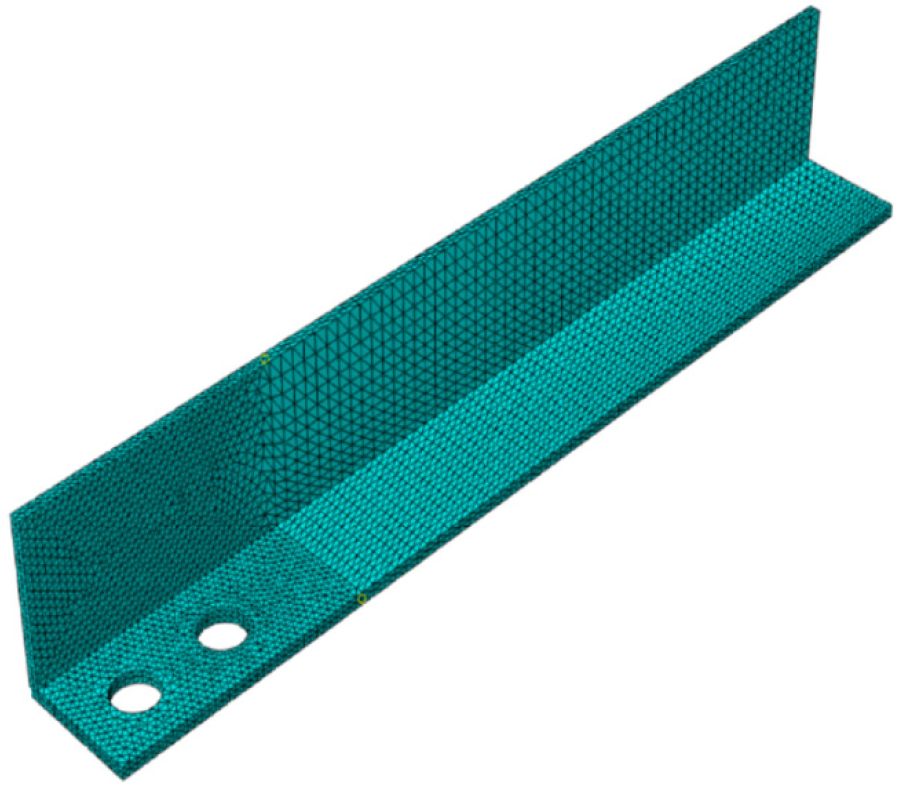### Angle section model before analysis.

Orbison, Wagner, and Fritz 1999 , worked on angle and tee sections angle section with 150*100* 7.82 mm dimensions were tested with two bolt connections and different edge distance of 50.8, 63.5 and 76.2 mm. The configuration of plates and bolt assembly is depicted in Figure 2. Gusset plates were fixed at both ends with two no of bolts on each side having diameter of 25.4 mm fitted snug-tight with drilled holes of diameter 27 mm. These specimen were tested in a testing machine with a capacity of 890 kN, which resulted in block shear failure of each specimen, rupture of tension plane along with inelastic shear deformation in gross shear plane was observed. In all experiments tension plane rupture started developing at bolt hole and followed till edge of section at maximum load.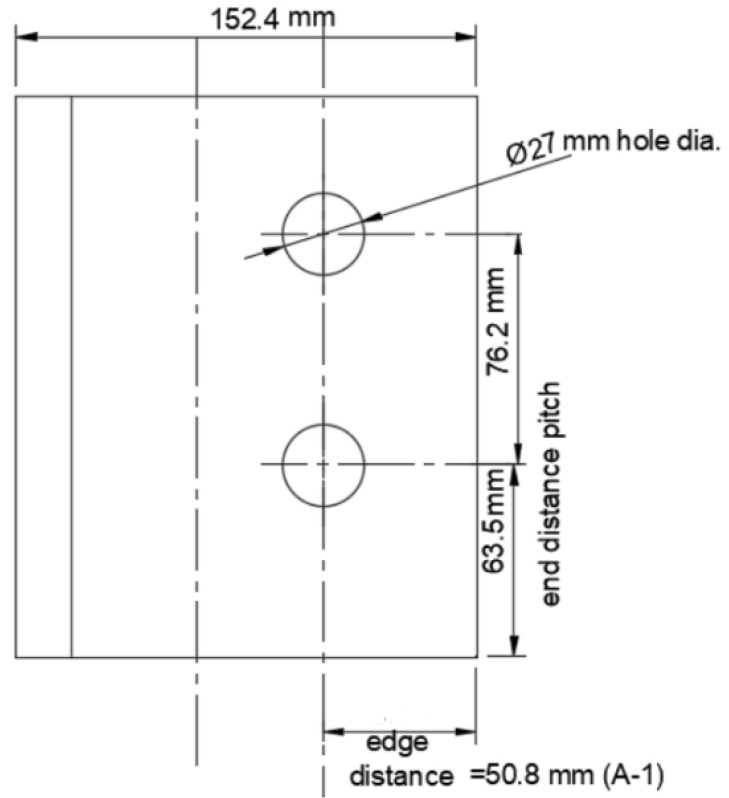### Angle section specimen geometry.

Yam, Jiang and Chung 2017 , conducted tests on angle section 85*65*6 connected to gusset plate at both ends through two bolts in line on each side with shorter leg. The diameter of bolt is 22 mm with holes of 24 mm and thickness of plate was 16 mm. Specimen was tested for tensile load applied on end of each gusset plate, block shear was observed in all the cases. Figure 3 shows the modelled specimen with connection geometry.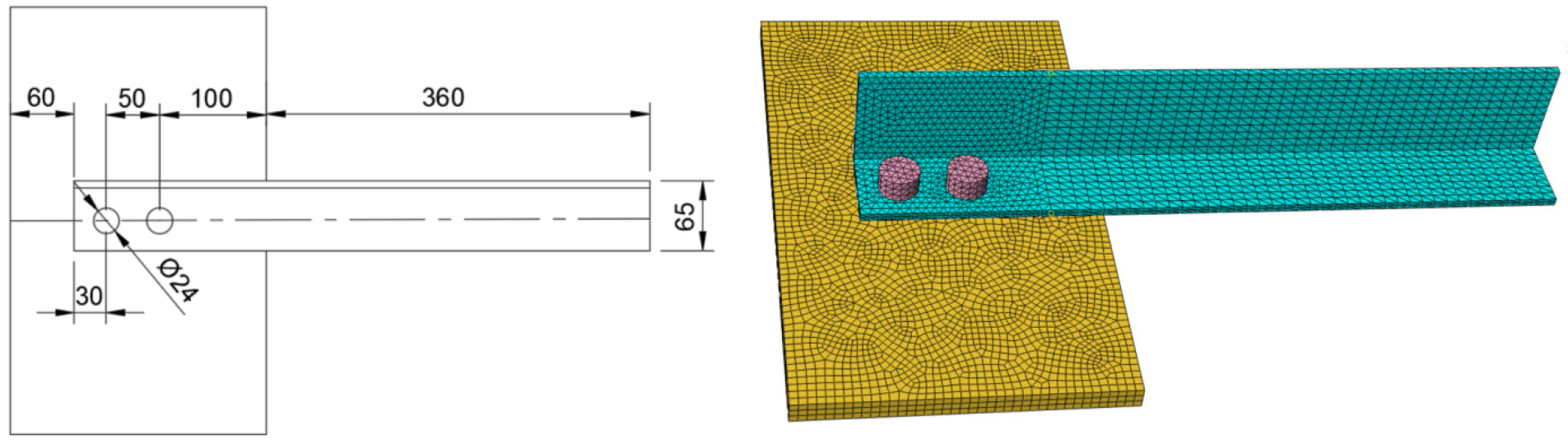### Section geometry in mm with modelling image.

Simulation of previous experiments for model validation

Previous mentioned studies were modelled with the current system and finite element mesh was prepaired. These models were analysed using the same method as adopted in original research, load capacity and failure pattern found through current modelling was recorded and checked with results published previously in original paper. Figure 1 shows model of undeformed angle section and Figure 4 shows a deformed angle section in finite element mesh model after analysis in software, necking of angle section and crumbling of gusset plate can be easily seen in the Figure 5. Comparison of finite element results with previous work on same samples has been shown in Table 1, which reflects that some samples were overestimated while for others the results were found to be unconservative. Professional factor (estimated load divided by predicted load) was found to carry out statistical analysis, which shows acceptable degree of consistency between predicted and experimental failure load. Professional factor of unity implies that predicted value is same as the experimental, while greater and lower values than unity gives underestimated and overestimated load capacities repectively. The comparison of modelling results in current study with previous researchers validates the modelling behaviour of current study, thus can be used to study specimen considered in this work and develop capacity prediction equations for block shear failure.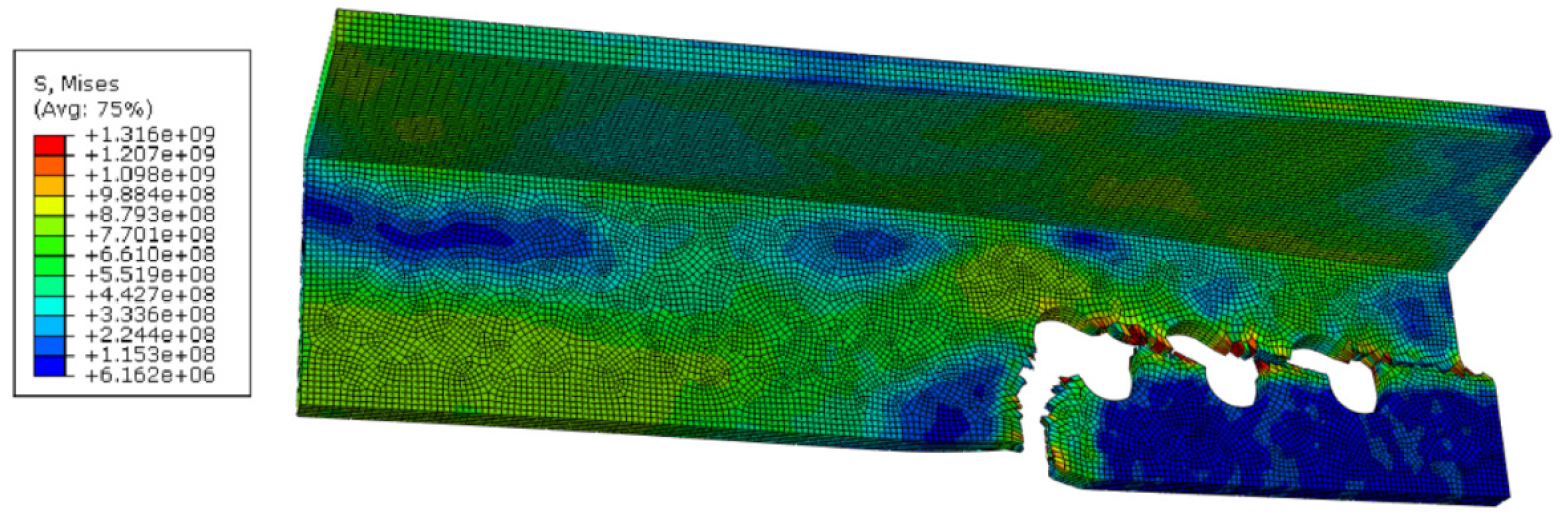### Block shear failure pattern of E758 angle section with two bolt connection.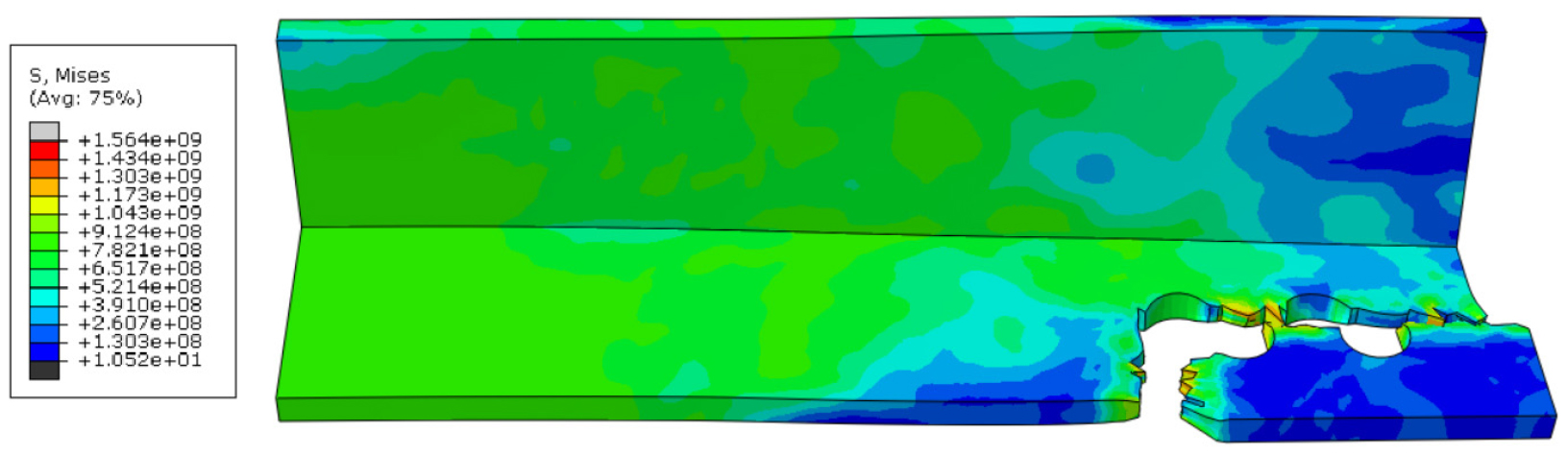### Block shear failure pattern of E758 angle section with 3 bolt connection.

#### Comparison of previous experimental failure loads with modelling study of present work

 Specimen designation Test failure load (kN) Modelling results Present Study Orbison, Wagner & Fritz (1999) A-1 362.1 383 A-2 444.8 431 A-3 500.0 527 Yam, Jiang & Chung (2017) A-Bt2 171.560 193 A-Bt3 222.740 234

High definition 3D finite element models were developed to exactly simulate and reproduce accurate results of experiments for all the reference specimens. Explicit dynamics mode of design with iso-parametric displacement based solid elements were assembled for creating connection components. Interaction mode selected was surface to surface explicit with contact property defined in tangential behaviour as penalty friction formulation (coefficient 0.2) and normal contact behaviour was hard contact. Also kinematic contact method was used for mechanical constraint formulation. Shaft of bolt and inner surface of holes were all in contact before loading and bolts were snug tight. Solid hexahedral shape of meshing with proportionate mesh scale was implied, general material property of steel were imported from the ABAQUS library which awarded suitable physical properties to the material. ABAQUS was adopted to figure out detailed finite element approach proposed for simulation. Tension force for displacement was simulated in explicit dynamics mode with element deletion, analysis of rupture and yielding till peak load value was recorded. The end reactions were simulated by restraining those nodes against displacement in perpendicular directions in the plane of plate which lie on circumference edge of each hole where bolts are in contact. Boundary condition for displacement in longitudinal direction is applied at opposite ends. Newton- Raphson method was used to trace the curve of load deflection response. Failure load is considered to be the point at which fracture initiates. Simulation showed necking around leading bolt hole is common in most of the sections, at failure load.

Current Experimental Program & Results

IS Steel Angle sections were fabricated to test in tension using Universal testing Machine prepared to test. Both equal and unequal angle sections were tested by fabricating with gusset plates on either side of angle section, connection between angle section and gusset plate was bolted with 18 mm bolts, having drilled holes. Thickness of gusset plate was greater than that of angle section in each type of specimen. Loading was applied at load displacement rate of 1mm/min which was continued until test specimen failed and separated into two parts. Curling was seen in most of the sections followed by necking of critical sections before rupture initiated. Specimen which failed abruptly due to eccentric loading, defective fabrication or some other reason, were eliminated from sample. In some of the specimen bending occurred in such a way that it caused conflicts with test set up, hence were discarded. Figure 6 shows an angle section being tested for tensile capacity in UTM.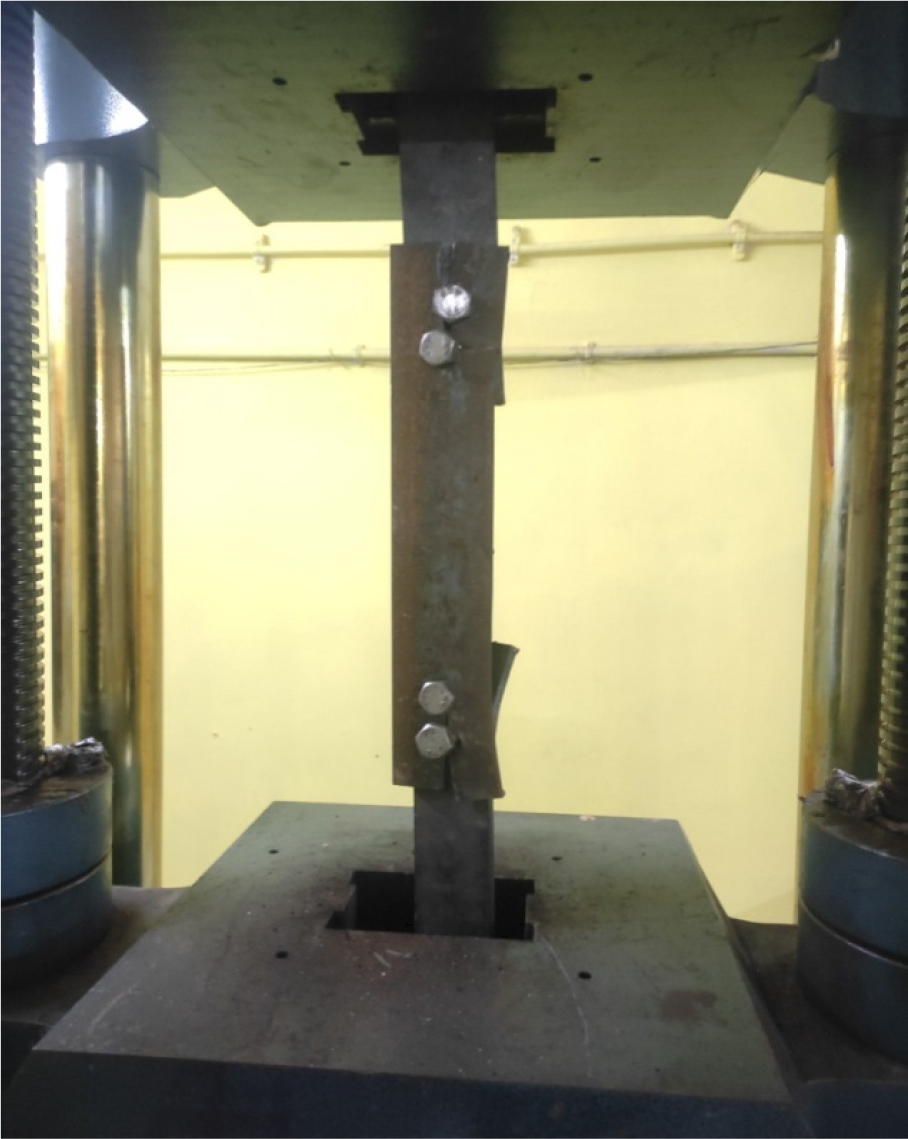### Angle section E758 in Universal Testing Machine for test in tension.

All the specimen failed in block shear depicting the pattern of failure involving rupture of tension plane and shear yielding of gross shear plane showing inelastic deformation. Rupture of tension plane initiated at bolt hole and propagated till the edge of specimen Figure 7 while yielding started along the length of shear plane, some cases depicted simultaneous yielding in both planes thereafter Figure 8 followed by rupture initiation in tension plane mostly in case of equal angle section. The results of experiment and calculated failure load has been compared in Table 2 and Table 3.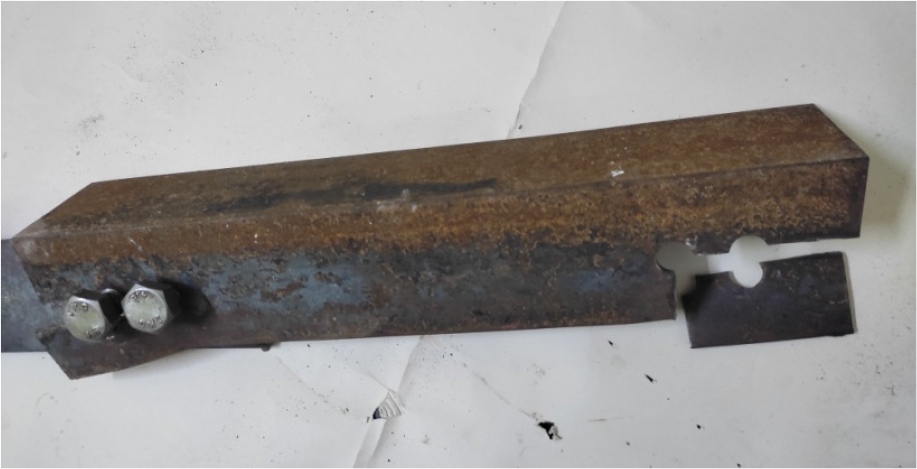### Angle E758 section failure with 2 bolts.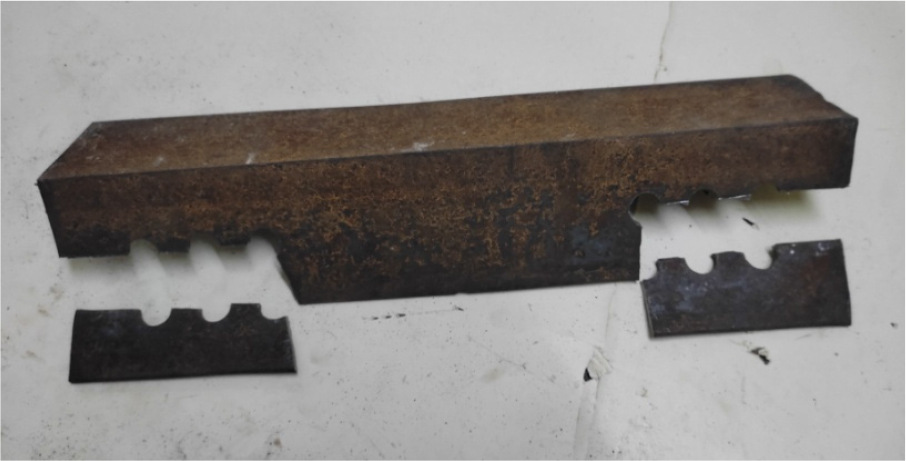### Angle E758 section failure with 3 bolts.

#### Equal angle section specimen results compared with theoretically calculated load

 Specimen Equal Angle Section Connected leg Calculated Failure load (kN) Experimental failure load(kN) E86 80X80X6 80 198.74 210 E88 80X80X8 80 264 278 E758 75X75X8 75 265 284 E96 90X90X6 90 198.7 214 E98 90X90X8 90 265 284 E118 110X110X8 110 264.9 291 E106 100X100X6 100 198 221 E108 100X100X8 100 264 295 E138 130X130X8 130 265 288

#### Unequal angle section specimen results compared with theoretically calculated load

 Specimen Angle Section Connected leg Calculated Failure load (kN) Experimental failure load (kN) U176 100X75X6 100 198 212 U716 75X100X6 75 198 201 U178 150X75X8 150 264 287 U718 75X150X8 75 264.9 271 U078 100X75X8 75 264 304 U708 75X100X8 75 264.982 283 U1276 125X75X6 125 198.7 220 U7126 75X125X6 75 198.737 191 U1278 125X75X8 125 265 283 U7128 75X125X8 75 264.982 269 U066 100X65X6 100 198.737 205 U606 65X100X6 65 198 196

Experimental and modelling analysis of current results indicates that equations proposed in the IS CODE 800-2007 mostly derives conservative value with substantial margin for some cases but in some cases of unequal angle section the result have been found to be overestimated. This may be due to the reason of greater length of connection in smaller sections and shear lag effect. The effect of Shear lag restrains the member to execute its full capacity strength as failure of connected leg occurs before complete stress distribution across the section.

Parametric Study

Parameters involved in the load carrying capacity of section influence failure strength in different ways, a parametric analysis was carried out to predict a new factor or constant which can be incorporated in existing equation for calculating block shear failure strength. To study the factors influencing block shear strength each factor involved had to be varied keeping others constant at a time, this was performed through modelling and no. of iterations. Pitch, end distance, edge distance, no. of bolts, bolt hole diameter are among the most significant parameters which cause difference in load carrying capacities. For all analysis same configuration of angle section and gusset plate with bolted joint was used.

It was found that net tension plane experienced ultimate stress at load of failure, where as gross shear plane yielded to high extent. These findings are supported by experimental results of samples which were tested physically, therefore theory of net section rupture and gross section yielding seems to be followed which will govern the design. A single equation which applies the same can be proposed with some modification for calculating of block shear. The modified equation should incorporate contribution of tension and shear planes and follows that failure occurs when net tension plane reaches ultimate stress value, yield stress of gross shear plane at which section fails only needs to be worked out then. The amount of yield largely depends on pitch and end distance which forms the length of connection, in this way gross section area calculation becomes important parameter, the calculation of gross shear area could be redefined or a factor can be associated with the same to find out the new equation. Numerical analysis would help in deriving the new equation and this tool can also be used for finding accuracy of present equation.

Common trend in a set of data reveals that for a constant yield to ultimate stress ratio, yielding of gross shear plane is affected by gross section area. Since gross section area is product of section thickness and length of connection, former being the constant length of connection remains to be critical . From close observation and mathematical calculations it was also seen that huge difference between net section length and gross section length led to an uneven distribution of stress in both net tension and gross shear plane are which are perpendicular. Probable cause of the same could be wrong consideration of gross section plane in which gross shear area is considered while only the section in between the bolt holes is subjected to shear yielding which could be identified from finite element analysis. Another noticeable stressed zone lied in the junction of both planes where radial length of junction bolt hole was considered in calculation of gross shear area, where as it was seen from finite element study that it was not subjected to stress.

However the current design through existing equations in IS 800:2007 and other codes give the results which are reasonable and mostly conservative with experimental failure loads, in case of greater edge distance connection design load is substantially underestimated probably due to the reason that net section also exhibits yielding before rupture initiates.

The analysis of observations in rupture pattern through modelling and series of values obtained in these set of test indicate that block shear capacity depends on value of yield stress generated in gross shear plane at the point when net section rupture starts developing in net section plane. Connection geometries, stress distribution and other parameters also reduce the block shear capacity with no contribution to shear plane. Hence new equation proposed shall be free from other factors and modification in gross shear plane area with some constant would give precise results.

Development of block shear load capacity prediction equations:

The aim of this work is to find a single equation and predict block shear load capacity, based on the numerical results, a number of iterations were performed to find out the relationship constant which can be introduced in the existing equation. Based on the evaluation of findings, equation is derived considering that net tension plane fails at ultimate stress and it is required to find the extent of shear in gross shear section plane at which section yields for that considered area of shear which has been marked in this study. Since there are many parameters involved in current expression, the new constant should not disturb their share of influence. Hence before proposing any alteration, it must be accounted that component of each factor in the equation is retained. Regression analysis and rounding off the coefficients helped in finding out solution for obtaining the value of constant.

##### (9)
$Tdb=[Avgfy/(1.3γm0)]*[≤ngthofconnection–[(no.ofbolts*diaofhole)]/(no.ofbolts**diaofhole)]+0.9Atnfu/γm1$

The above equation can be rewritten as

##### (10)
$Tdb=[Avgfy/(1.3γm0)]*[(Lc-Nb*ϕ)/(Nb*ϕ)]+0.9Atnfu/γm1$

$Lc$ = length of connection = [(no. of bolt holes* pitch) +end dist.+ (0.5*ϕ)]

$NB$ = no. of bolt holes

ϕ = diameter of bolt hole

Where, $Tdb$ Block shear strength

$Avg$ and $Avn$ are minimum gross and net area in shear along bolt line parallel to external force, respectively

$Atg$ and $Atn$ are minimum gross and net area in tension from the bolt hole to the toe of the angle, end bolt line, perpendicular to the line of force, respectively

$fu$ = Characteristic ultimate tensile stress

$fy$ = Characteristic yield stress

$γm1$= Partial safety factor against ultimate stress

$γm0$= Partial safety factor against yield stress and buckling

The accuracy of predicted equation was assessed by comparing with the experimental and finite element modelling results. Comparison of calculated load from developed equation and modelling results are shown in Table 4 and Table 5, statistical measures of predictions are given in Table 7. Statistical measures show that predicted block shear capacity is in concurrence with experimental and modelling results. In order to find out the accuracy of proposed equation chi-square test was performed which accepted the null hypothesis proving that predict values are same as observed values. Results of chi-square test have been tabulated in Table 6. The average professional factor (ratio of experimental load to predicted value calculated by derived equation) is nearly 0.96 and standard deviation of 3%. Variation of 15% in strength which may be under or overestimated can be noticed from professional factors. The recorded values show that under strength upto 14% was seen in a specimen smaller section and short length connection, these type of cases can be referred to be calculated through conventional procedure. Overstrength was predicted for unequal small angle section cases with long connections, since the values were conservative such sections can be accounted by this equation. The failure pattern for some specimen indicate failure mode in which combined effect of net section and block shear failure occurred also some cases failed by continued tearing at an angle from shear plane after the tension plane rupture, in such cases the developed equation failed to predict results accurately.

#### Equal angle section specimen modelling results compared with values obtained from proposed equation

 Specimen Equal Angle Section Connected leg Finite element modelling value (kN) Value from Developed equation (kN) E86 80X80X6 80 206 219.6 E88 80X80X8 80 267 292.8 E758 75X75X8 75 277 292.8 E96 90X90X6 90 209 219.6 E98 90X90X8 90 302 292.8 E118 110X110X8 110 309 292.8 E106 100X100X6 100 297 219.6 E108 100X100X8 100 312 292.8 E138 130X130X8 130 321 292.8

#### Unequal angle section specimen modelling results compared with values obtained from proposed equation

 Specimen Unequal Angle Section Connected leg Finite element modelling value (kN) Failure load from Developed equation(kN) U176 100X75X6 100 217 219.6 U716 75X100X6 75 210 219.6 U178 150X75X8 150 279 292.8 U718 75X150X8 75 262 292.8 U078 100X75X8 75 284 292.8 U708 75X100X8 75 271 292.8 U1276 125X75X6 125 211 219.6 U7126 75X125X6 75 201 219.6 U1278 125X75X8 125 302 292.8 U7128 75X125X8 75 289 292.8 U066 100X65X6 100 202 219.6 U606 65X100X6 65 191 219.6

#### χ² (Chi) square test values

 Total (χ² calculated value) 15.85 Degree of freedom (df) 20 χ² critical value (from chi square distribution table) 31.41 χ² critical value > χ² calculated value Therefore, null hypothesis is accepted, and there is no difference between predicted and observed value

#### Professional factors range

 Mean 0.96 Standard deviation 0.04 Maximum 1.04 Minimum 0.87

Conclusion

A high definition finite element model was developed to carry out analysis of block shear failure in steel angle tension members, this method of modelling was validated with experimental investigations published by Orbison, Wagner, and Fritz 1999 and Yam, Jiang and Chung 2017, who conducted tests on angle section 85*65*6 connected to gusset plate at both ends through two bolts in line on each side with shorter leg. The diameter of bolt is 22 mm with holes of 24 mm and thickness of plate was 16 mm. Specimen were tested for tensile load applied on end of each gusset plate, block shear was observed in all the cases.

The result of physical experiments in current work was also scrutinized to verify modelling output and found satisfactory. Such a model is helpful in investigating block shear failure in other angle sections, plates and tees as this model can predict failure loads to an acceptable degree of precision. Figure 9 and Figure 10 shows the concurrency of results between proposed equation and experimental values. Parameters associated with block shear failure of angle section in tension were studied to find out their share of influence and identify a constant which could be introduced in proposed equation to calculate block shear failure capacity. The analytical findings lead to the development of an equation which was checked with result of experiments.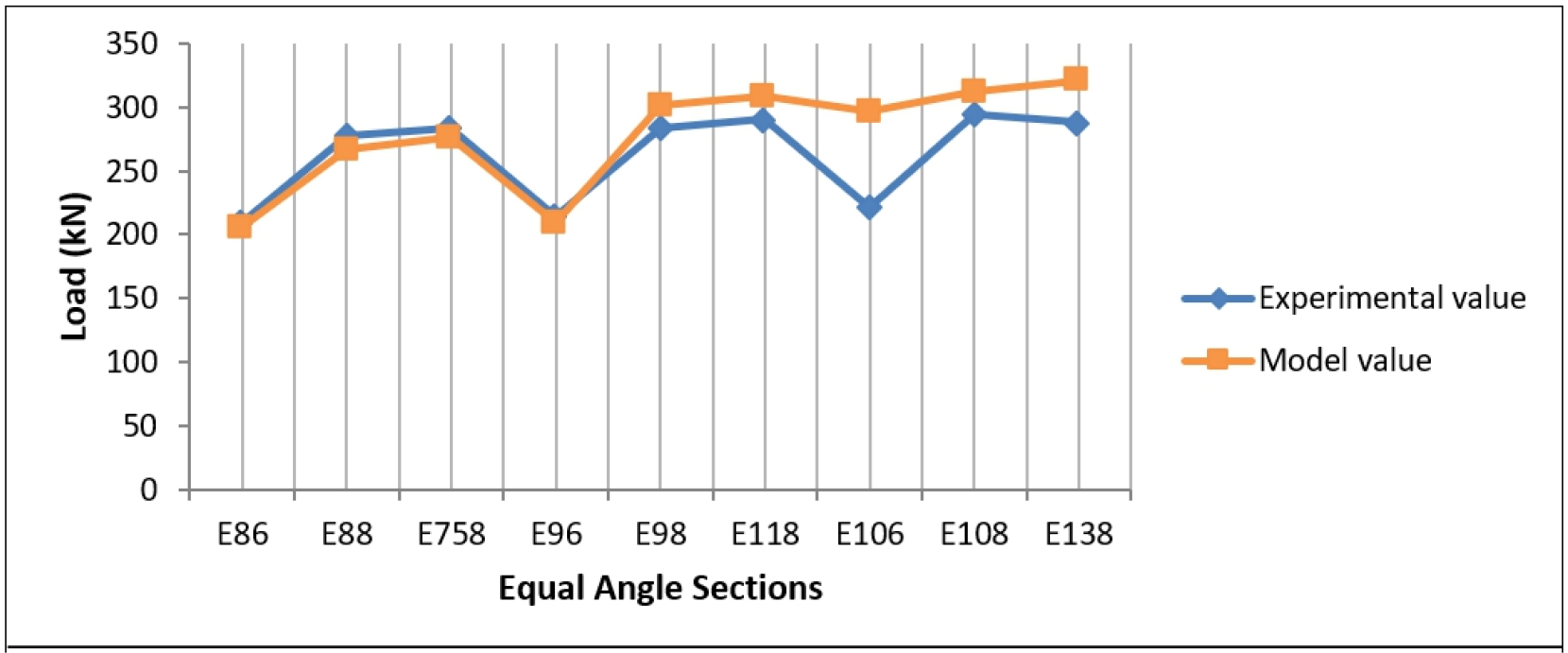### Capacity curve of Modelling results compared with physical experiment value of corresponding failure load in equal and unequal angle section.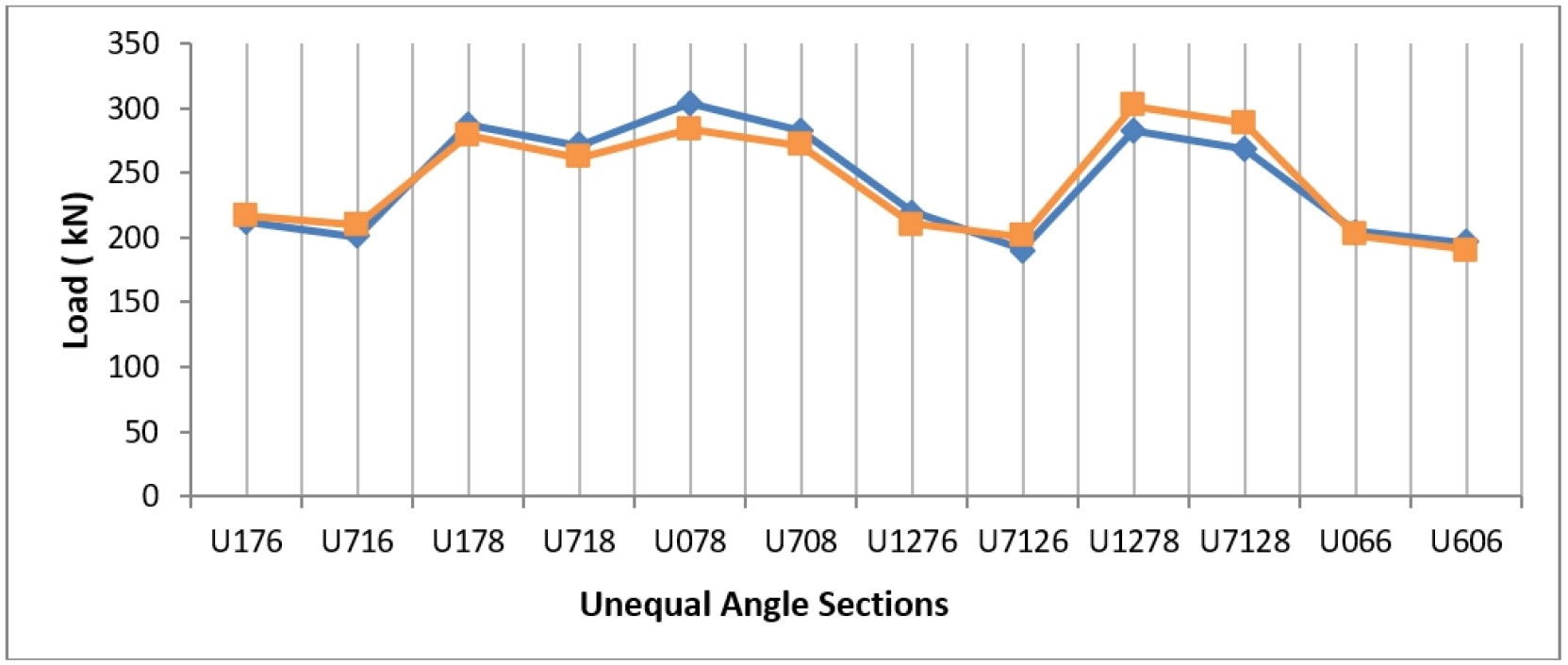### Capacity curve of Modelling results compared with physical experiment value of corresponding failure load in equal angle section.

The following can be concluded from this study.

The equation proposed derives strength value with fair precision, this equation could be an alternative to conventionally prescribed codal equations.

Block shear capacity is greatly affected by area of shear plane which yields under loading, this area of section need to be calculated by reduction for radial length of lead and last bolt hole along the length of connection.

Zone of stress at the junction of net tension plane and gross shear plane plays a significant role in strength capacity of connection due to large difference between the length of net tension plane and gross shear plane.

Further Scope of Work

Further investigation is required for the factors which have not been considered in this study :

Bock shear failure for tension members of angle section was considered in this study, other section such as Tees, channels and plates shall be studied.

Type of failure studies is restricted to block shear in this work which can be further extended to other types as it was found during experiments that some section failed due to combination of block shear and net section which has been termed as partial net section rupture , equations for such type of failure may be derived.

The equation proposed works for section with non staggered type of connected bolts, therefore staggered bolting and multiple bolt line cases can be studied hereafter.

Von Mises yield criterion is assumed to follow throughout the stress-strain deformation region, this failure mechanism can also be studied using Tresca yield criterion which predicts narrower elastic region .

Developed equation is derived on the basis that ultimate load is experienced by the net tension plane and shear stress of yield in gross shear plane is required to be found, local strains generated which eventually lead to same stress have not been accounted which needs to be studied and modified capacity equations can be formulated.

## References

1
M.C.H. Yam, B. Jiang, and K.F. Chung, Numerical studies on block shear failure of bolted high strength steel angles. The 2017 world congress on Advances in Structural Engineering and Mechanics (ASEM17), llsam (Seoul), Korea. (2017).
2
C.O. Rex and W.S. Easterling, Behavior and Modeling of a Bolt Bearing on a Single Plate. Journal of Structural Engineering. 129(6) (2003), 792. DOI: 10.1061/(ASCE)0733-9445. 10.1061/(ASCE)0733-9445(2003)129:6(792)
3
R. Puthli and O. Fleischer, Investigations on bolted connections for high strength steel members. Journal of Constructional Steel Research. 57(3) (2001), pp. 313-326. 10.1016/S0143-974X(00)00017-1
4
P. Prabha, S. Arul Jayachandran, M. Saravanan, and V. Marimuthu, Prediction of the tensile capacity of cold formed angles experiencing shear lag. Thin-Walled Structures. 49(11) (2011), pp. 1348- 1358. 10.1016/j.tws.2011.06.003
5
V.F. de Paula, L.M.Bezerra, and W.T. Matias, Efficiency reduction due to shear lag on bolted cold-formed steel angles. Journal of Constructional Steel Research. 64(5) (2008), pp. 571-583. 10.1016/j.jcsr.2007.10.008
6
J.G. Orbison, M.E. Wagner, and W.P. Fritz, Tension plane behavior in single-row bolted connections subject to block shear. Journal of Constructional Steel Research. 49(3) (1999), pp. 225-239. 10.1016/S0143-974X(98)90172-9
7
A. Mohamed Nainakkan Subuhan and Dr.P. Jayabalan (2012) Block Shear Analysis of Tension Member. Proceedings of International Conference on Advances in Architecture and Civil Engineering (AARCV 2012), 21st - 23rd June 2012, Paper ID SAM190, Vol. 1.
8
L.H. Teh and D.D.A. Clements, Mechanisms of Block Shear Failure of Bolted Connections. International Specialty Conference on Cold-Formed Steel Structures 3. (2012). Available at: https:// scholarsmine.mst.edu/isccss/21iccfss/21iccfss-session9/3.
9
L.H. Teh and G. Deierlein, Effective Shear Plane Model For Tearout And Block Shear Failure of Bolted Connections. Faculty of Engineering And Information Sciences - Papers: Part B. 1507. Engineering Journal-american Institute Of Steel Construction, 54(3) (2017), pp. 181-194. Available at: https://ro.uow.edu.au/eispapers1/1507.
10
M. Gupta and L.M. Gupta, Evaluation of stress distribution in bolted steel angles under tension. Electronic Journal of Structural Engineering. 4 (2004), pp. 17-27. 10.56748/ejse.438
11
E. Bernatowska and L. Ślęczka, Experimental and Numerical Investigation into Failure Modes of Tension Angle Members Connected by One Leg. Materials. 14(18) (2021), 5141. DOI: https://doi. org/10.3390/ma14185141. 10.3390/ma1418514134576363PMC8470118
12
J.R. Dhanuskar and L.M. Gupta, Experimental Investigation of Block Shear Failure in a Single Angle Tension Member. International Journal of Steel Structures. 20 (2020). DOI: https://doi.org/ 10.1007/s13296-020-00398-2. 10.1007/s13296-020-00398-2
13
M.D. Elliott, L.H. Teh, and A. Ahmed, Behaviour and strength of bolted connections failing in shear. Journal of Constructional Steel Research. 153 (2019), pp. 320-329. 10.1016/j.jcsr.2018.10.029
14
B. Jiang, M.C.H. Yam, K. Ke, A.C.C. Lam, and Q. Zhao, Block shear failure of S275 and S690 steel angles with single-line bolted connections. Journal of Constructional Steel Research. 170 (2020),106068. 10.1016/j.jcsr.2020.106068
15
C. Topkaya, A finite element parametric study on block shear failure of steel tension member. Journal of Constructional Steel Research. 60(11) (2004), pp. 1615-1635. 10.1016/j.jcsr.2004.03.006
16
K.E. Barth, J.G. Orbison, and R. Nukala, Behavior of steel tension members subjected to uniaxial loading. Journal of Constructional Steel Research. 58(5-8) (2002), pp. 1103-1120. 10.1016/S0143-974X(01)00082-7
17
A.P. Singulani, Advanced Methods for Mechanical Analysis and Simulation of Through Silicon Vias. Dissertation, TU Wien - Institute for Microelectronics, Veinna, Austria. 2014. Available at: https:// www.iue.tuwien.ac.at/phd/singulani/diss.html.